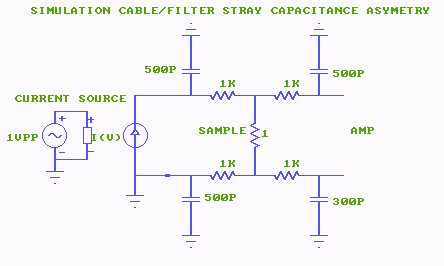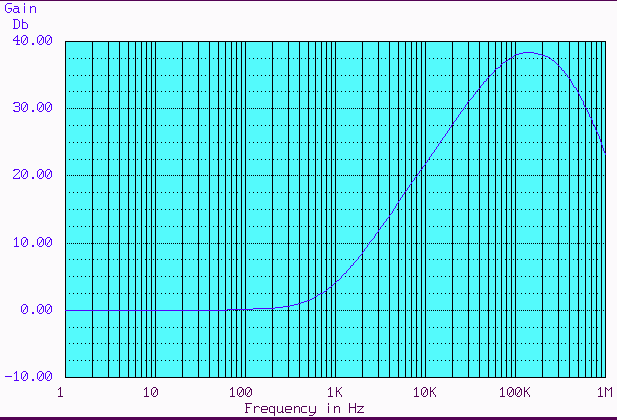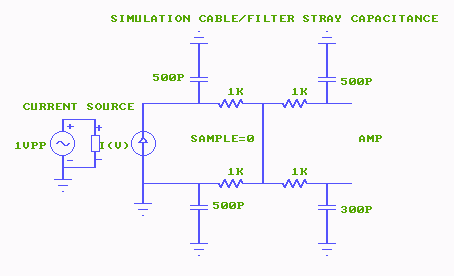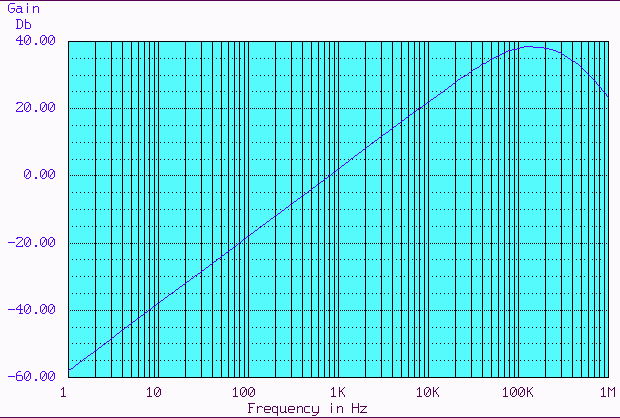QT designed instrumentation -measure-cable capacitance

 Wires to the sample have a capacitance to ground (shield) unequal capacitances can create additional differential signals Here at 10KHz ,the error is 10 times the signal to be measuredError signal created by unequal lines to the sample, simulated amp input signal 0db=signal level created by sampleWires to the sample have a capacitance to ground (shield) unequal capacitances can create a differential signal where there is actually no sample related signal present, Rsample = 0 ohmError signal created by unequal lines to the sample,simulated amplifier input signal sample = 0 ohm, no signal from sample